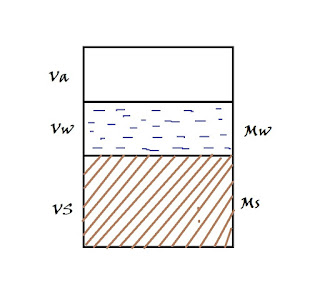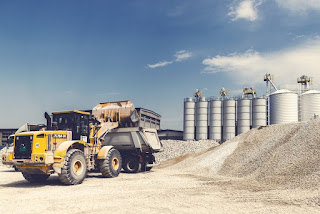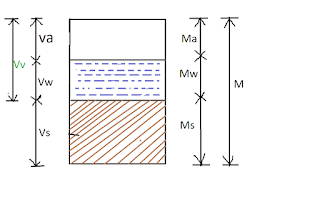# Engineering

Find The exclusive collection of Engineering on YourHop.com, Do not forget to Comment and Share if below Engineering is helpful for you.

### Relationship Between [Void Ratio], Specific Gravity / [Degree of Saturation]In three phase diagrame, we see volumetric relations and volume mass relation, further, thair is Relationship between void ratio specific gravity and degree of saturation.which important in solving the numerical problem and for a better understanding of the chapter.  Fig Show a three phase diagram, the left side of which shows Volume and right side show mass respectively for air-water solid. We know Density ρ = mass / Volume  also this can be written as  mass = Density x Volume from above we say the mass of water = Density of water x Volume of water = ρw x Vw Now Mass of solid = density of solid x Volume of Solid , But We don't know the density of solid but we know Specific Gravity (G) = Density of solid ρs / Density of Water ρw , from this we can say Density of solid is ρs = G x ρw Put these density value in Mass formula M= ρv our diagram now look like this (second three phase diagram) Also, we know water content = Degree of saturation S = volume of water Vw  / volume of voids

### [SOIL PERMEABILITY] Definition / Test to Find Permeabilitywe discuss whereas types of water in soil on lesson capillary water , one of them is free water ( the water which flows from one point to other by the influence of gravity ). this free water gives permeability to the soil. we also know soil mass contain pores or holes. if these porous are interconnected its called interstices, free water flow throw it from one place to other places on soil mass.  Permeability of soil  From the above discussion, we can say the property of soil which permits flow of water on it Called permeability. However the velocity of water or say permeability may depend upon how much width of these interstices, for example, coarse grain soil give high permeability effect as compare to fine grain soil, because the particle gap is large in case of coarse grain as compared to fine grain. generally, all type of soil is permeable, if the soil is a very low permeability its called impervious soil. permeability is very important engineering property. it helps in design f

### [SOIL STABILIZATION] Definition | TypesWhen soil Available at a construction site is not Suitable For our construction Due is its low-level Property, then its required to improved engineering property (like Permeability, Shear strength ) and make our soil ready for construction, all these processes of improving soil is called Soil stabilization. the stabilization of any soil includes the term compaction, drainage, and Preconsolidation mean these cover in the stabilization process. With the help of the soil stabilization process, we quickly make good workability condition on any type of soil. specially trafficable area and in case of emergency military movement. Method of Soil Stabilization There are lots of soil stabilization technique used in modern days which are following Soil stabilization with cement Mechanical Stabilization of Soil Bituminous  Stabilization Soil stabilization using lime Chemical Stabilization of Soil Fabric and Geotextile Stabilization of Soil Thermal Stabilization of Soil Reinforced Stabilization of

### How [Capillary Action of Water] Movement Work in SoilCapillary Water When You Talking About Important Topic in Soil mechanics and Also in other Engineering Subject Capillary Water Surface tension is one of the most important Topic. This is lesson 1 in which we talk about only  Capillary water basic and surface tension. So the Question 1  is What is Capillary Water? Before Discussing Capillary water it Very important to see this Graph of the type of soil water. Free Water In soil -  these water flows from one point to other were different of total head Present. mainly reason of this is due to an influence of the gravity. Now we know the particular space in soil is very low so this water is a laminar Flow type. ( maybe non-laminar in Case of coarse soil) Free Water Give the Permeability Effect in soil Held Water-  it does not move under the influence of the gravity, basically held water is retained in the Pores of the soil. Structure Water   - This water is Part of the crystal structure of the mineral of the soil. it chemically combined

### Relationship Between Void Ratio and Porositythe porosity and void ratio both indicates the void hole in a soil mass but it a different as we discuss in three phase diagram 👈 of volumetric relation . the voids ratio define how much voids present as compared to total solids in soil mass, where porosity say how much voids as compared to total volume of soil mass, in void ratio if the void increase in soil mass only top value change. but in porosity both top and bottom value change . 👈 there is a relation between void ratio and porosity which very important in soil problem discuss below.  The relation between porosity and void ratio we know formula of void ratio (e) = Vv / Vs in which Vv = Volume of Voids and Vs = Volume of Solids Porosity formula (n) = Vv / V  Vv = Volume of Voids and V= Total Volume of soil mass. first letter V represent volume and second letter a= air, W= water S= solid also the volume of voids = Vw + Va Now finding the relation between (e) a

### What is [FROST HEAVE] | Frost Boil Definition, Prevention and SolutionsIn the previous lesson we study about the capillary water rise in the soil , you can relate Frost heave to capillary water for better understanding. we know due to capillary action, water rise in the soil above the water table. and if the atmospheric temperature is below freezing point this water has a chance to convert into ice, now action begins. there is a fact between water and ice - when water change to ice its volume increase. this fact responsible for the heave. Frost Heave when water freeze in soil its volume increase and try to expand the soil which gives an undulation heave to the ground surface this phenomenon called frost heave. heave has also the power to lift the small structure. which not good for any structure safety. Now we know fine grain soil has high capillary rise as compared to coarse grain soil it does not mean every fine grain soil give frost heave. another factor called permeability is responsible for the frost heave action. some fine grain soil like Silt, fine

### What is [BULKING OF SAND] | How its Different than Slaking of ClayBulking of sand is the phenomenon in which the volume of sand increases, mainly due to capillary action or adding some specific value of water content (ex- 4-5%) to dry sand. we know sand is not plastic in nature, assuming dry sand placed on the ground where the capillary action occurred. Due to this action water start uplift throw sand particle. and the sand becomes damp. in this damp sand, cohesion(attraction of water molecule) develop throwout capillary rice. this attraction prevents sand particle to taking a stable position. because in the previous lesson we know capillary water produced upward pressure. from the all above definition we say the volume of the sand increase due to damping, this increased volume is called bulking of sand. also, note that if you increase more water than a reasonable amount, capillary action destroyed and volume decrease. you can check bulking of sand at home by adding some water to sand, bulking is maximum at water content around 4-5% Slaking of clay

### What is degree of Saturation definition, Formula | Equation

For Proper Understanding Of terms, we Highly recomend to see these Past Before any Reading Three Phase Diagram of Soil Void Ratio of soil Porosity of Soil Degree of saturation - Its Defined  how Much water  present in the pores of soil as compared to total voids volume in the soil mass. Denoted by (S) and the formula is  S = Vw / Vv The value of s is written in percentage, when a soil mass is fully saturated S = 100% if a soil mass completely dry its value is 0.

### What is Porosity of soil definition, Formula & Equation

For Proper Understanding Of terms, we Highly recommend to see these Topics Before any Reading Three Phase Diagram Void Ratio of soil Degree of Saturation Porosity of soil - Porosity refers to little hole or pores in the soil mass. these hole filled by water and air. porosity look same as void ratio by definition but the difference between these are porosity defines how much is total holes as compared to total volume. But voids ration define total hole as compare to solids. if you visualize then see porosity not go above 1 as compare to voids ratio. because voids volume never increases above total volume. Porosity is Denoted by (n) and is formula is e = Vv/V there is a one most important 👉 relation between void ratio and porosity 👈 . which used in many soil mechanics problem. its recommended  to see that relation the denseness of soil measure from porosity and also from void ratio because as porous in soil decrease the denseness of soil increase. if pore value is more then soil bec

### What is Air content, Air voids Definition, Formula / EquationFor Proper Understanding Of terms, we Highly recommend to see these Past Topics Before any Reading Three Phase Diagram Void Ratio of soil Porosity of Soil Degree of Saturation Air Content - Defined how Much volume of air present in the pores of soil as compared to total voids volume in the soil mass. the value of air content is zero when the soil is fully saturated because of no air present in the fully saturated soil. Air content (ac) = Va / Vv Percentage air voids - its defined how much percentage of air is present as compare to total volume in a soil mass. denoted by (na) = Va / V The relation between air content and percentage Air Voids. from above relation, we can say percentage air voids is equal to porosity multiply air content.